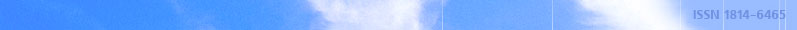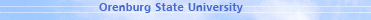january 20, 2022 RU/EN
 Headings of Vestnik Pedagogics Psychology Other

 Search Author Article

Shevelenko V.D., Lukoyanov V.A.
USING OF THE ABEL TRANSFORM TO SUM TRIGONOMETRIC POLYNOMIALS [№ 4 ' 2015]
Currently, the spectral signal analysis commonly used digital filter based on DSP with the input of ADC (analog-to-digital converter), which implements the ordinary or fast discrete Fourier transform. Speed digital filter determines the width of the analyzed spectrum of the measured process and the ability to process data in real time. Most characteristic for the speed of digital filters based on dedicated processors fast Fourier transform, multiplying the matrix comprising a conveyor with parallel data processing. However, parallelization of computing operations leads to a nonlinear increase in the volume of equipment. Therefore, despite the rather significant process in improving the performance of digital element base, the computing speed of the need to perform parallel-serial number of multiplications is relatively increased substantially less, which leads to inefficient use of components. The upper cutoff frequency spectrum of the signal that can be analyzed not exceed a few dozen kilohertz, at a number of points 1024, and accordingly, the number of harmonics equal to 512. In solving the problems of filtration, requiring a large number of multiplications, to increase the speed of signal processing by the discrete Fourier transform is needed transition to digital-to-analog and analog multiplier with a maximum parallelization of the encoding and signal processing, and implementation of these operations as much as possible in the devices. This need is caused by the fact that the expansion of the frequency spectrum of the analyzed dimensions, current consumption and the cost of digital "Fourier-processor" increases dramatically.The article discusses the possibility of using the Abel transformation to summation of trigonometric functions. It shows some of the common sum of the coefficients. It considers conclusions, under which it is possible to exist special cases, when the using of second Abel transformation form to summation of trigonometric functions will be more appropriate.

Frolov S.S., Shevelenko V.D., Gusarov A.A.
APPROXIMATION METHOD OF SINUSOIDAL EQUAL-AMPLITUDE POLYNOMIAL [№ 9 ' 2006]
One of approximation methods of equal-amplitude trigonometrical polynomials having equal permanent discrete spectrum, - approximation method of sinusoidal polynomial is suggested in this article. Analytical expressions for discrete harmonic spectrum of approximate function are given and its determinant characteristics – coefficient of parasite spectrum harmonics and unevenness of harmonics amplitudes at working sphere of spectrum are calculated in this work.

Frolov S.S., Shevelenko V.D., Burkova E.V.
METHOD OF APPROXIMATION OF EQUAL AMPLITUDE POLYNOMIAL [№ 5 ' 2006]
Low effectiveness of modern measuring signals using for measuring of frequency characteristics in the sphere of infralow frequency is shown in this article. New signal – trigonometrical polynomial with equal discontinuous spectrum and method of its approximation are suggested here. Analytical expressions of discontinuous harmonic spectrum of approximation function are got. Methodic of approximation errors appraisal in the form of stray spectrum harmonics coefficient and unevenness of amplitude spectrum at working time is offered in this work.

Gusarov A.A., Kutuzov V.I., Shevelenko V.D.
INCREASE OF DIAGNOSIS EFFECTIVENESS OF ROD OIL AGGREGATE [№ 2 ' 2006]
Expressions for harmonic constituents of effort on pump piston through corresponding signals of effort and way, measured on surface were got on the base of considering of rods columns of pumping units as mechanical communication channel giving information in the form of deformation waves from pump piston to the point of rods hanger with spectral method. Form deflection of plunger dynamometer card from "sample", caused with classes of rod oil aggregate "ШНУ" condition, are determined with measuring of amplitude modulation depth of harmonics, having maximal susceptibility to measuring of diagnosticated class of ШНУ condition.

Raimova A.T., Kutuzov V.I., Vakulyuk V.M., Shevelenko V.D.
METHOD OF PERIODIZATED SIGNAL RECREATION AT ACCEPTED DISCRETE READINGS [№ 1 ' 2006]
Method of periodizated signal recreation at accepted discrete readings based on decomposition of periodizated signal in row of mutually-orthogonal functions, making "nucleus Dirikhle", which is impact function of ideal discrete filter, is suggested in this article. It is shown that high level of metrological indexes of method is determined with obtainable relative instability of carrier frequency oscillations using for forming of uniform-amplitude polynomials in the form of amplitude-modulated oscillations. The method allows fulfilling signal recreation in the rate of readings receipt.

Kvitek E.V., Kutuzov V.I., Shevelenko V.D.
INCREASE OF SPEED OF INFORMATION HARMONICS PARAMETERS DETERMINATION BY THE METHOD FURIE OF GROUP TRANSFORMATION [№ 1 ' 2005]
Expressions for corresponding group signals, allowing to change the character of transformations in the process of realization of the direct return Furie transformations, which allowes to decrease frequency of discretization in comparison with Nayquist frequency for ranges of lower and middle frequencies, are given on the base of signals presentation as a group of frequency components, amassed in the are of lower frequencies, higher and middle friquencies. It allows to reduce number of calculating processes in comparison with needed on the base of quick Furie transformation.

Kvitek E.V., Shevelenko V.D
IDENTIFYING PARAMETERS OF TECHNOLOGICAL PROCESSES APPROXIMIED BY SPLINES IN FREQUENCY FIELD [№ 1 ' 2003]
The article analyses the possibility of identifying technological processes more accurately by means of presenting them by splines in frequency field instead of a time field.

Ye.V.Kvitek, V.N.Tarasov, V.D.Shevelenko
FILTRATION OF MEASURING SIGNALS BY THE POLYNOMIAL ORTHOGONALIZATION METHOD [№ 3 ' 2000]
Filtration spectral method of measuring signals is considered in this article. This method is based on the possibility of signal transformation contraction by means of changing harmonic function basis into Kernel Dirihle basis.

Shevelenko V.D., Shevelenko D.V, Kvitek E.V.
FILTRATION OF MEASURING SIGNALS BY MEANS OF FORMING THE QUOTIENT SUMS OF FURJE ROWS [№ 1 ' 1999]
This article describes the spectral method of filtration of measuring signals founded on the possibility to reduce (to cancel) the volumes of transformations over a signal by means of transition from the basis of harmonic functions to the basis in the form of Dirichle nucleus. Correlations providing the realisation of the filtre property of orthorationing basis by means of the reproducing the Dirichle nucleus in the form of the amplitude-modulated oscillation were received. The authors show the possibility of the practical realisation of the filtre device and assess its errors.Editor-in-chief Sergey Aleksandrovich MIROSHNIKOV

 © Электронное периодическое издание: ВЕСТНИК ОГУ on-line (VESTNIK OSU on-line), ISSN on-line 1814-6465 Зарегистрировано в Федеральной службе по надзору в сфере связи, информационных технологий и массовых коммуникаций Свидетельство о регистрации СМИ: Эл № ФС77-37678 от 29 сентября 2009 г. Учредитель: Оренбургский государственный университет (ОГУ) Главный редактор: С.А. Мирошников Адрес редакции: 460018, г. Оренбург, проспект Победы, д. 13, к. 2335 Тел./факс: (3532)37-27-78 E-mail: vestnik@mail.osu.ru 1999–2022 © CIT OSU